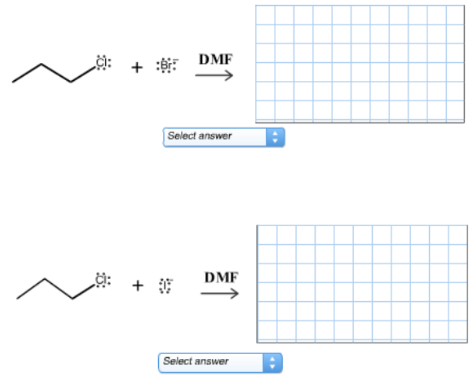Organic Chemistry Practice Problems Substitution Comparison Practice Problems Solution: Consider the following pair of reactions. Predict ...

🤓 Based on our data, we think this question is relevant for Professor Crowe's class at LSU.

# Solution: Consider the following pair of reactions. Predict the type of substitution mechanism, predict which reaction of the pair will occur at the faster rate, and draw the correct organic product.

###### Problem

Consider the following pair of reactions. Predict the type of substitution mechanism, predict which reaction of the pair will occur at the faster rate, and draw the correct organic product.Substitution Comparison

Substitution Comparison

#### Q. A set of three nucleophilic displacement reactions is shown below:Which reaction (A, B, or C) proceeds the fastest?A set of three nucleophilic displac...

Solved • Tue Mar 17 2020 09:24:19 GMT-0400 (EDT)

Substitution Comparison

#### Q. Consider the following pair of reactions. Predict the type of substitution mechanism, predict which reaction of the pair will occur at the faster rate...

Solved • Fri May 03 2019 17:01:11 GMT-0400 (EDT)

Substitution Comparison

#### Q. Consider the following pair of reactions. Predict the type of substitution mechanism, predict which reaction of the pair will occur at the faster rate...

Solved • Fri Apr 26 2019 19:22:38 GMT-0400 (EDT)

Substitution Comparison

#### Q. Consider the following pair of reactions. Predict the type of substitution mechanism, predict which reaction of the pair will occur at the faster rate...

Solved • Thu Apr 25 2019 21:05:32 GMT-0400 (EDT)